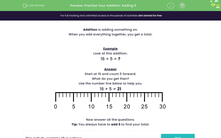Comprehensive & curriculum aligned

In this worksheets, students practise adding the number 5 to other numbers in the range 0 to 20.Key stage:  KS 1

Curriculum topic:   Number: Addition and Subtraction

Curriculum subtopic:   Add and Subtract to 20

Difficulty level:Worksheet Overview

When you add everything together, you get a total.

Example

16 + 5 = ?

Start at 16 and count 5 forward.

What do you get then?

16 + 5 = 21